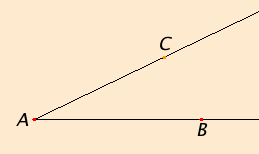# Definition 9

And when the lines containing the angle are straight, the angle is called rectilinear.

## Guide

 This continues the previous definition of angle. Nearly all the angles that appear in the Elements are rectilinear as is the illustrated angle BAC. Angles usually are named by three points, the middle point the vertex of the angle. When there is no ambiguity it is sufficient to name the angle by its vertex, in this example, A.#### Angles as magnitudesAs treated by Euclid, rectilinear angles are magnitudes that can be added together. When they are angles that share a side, and their sum is less than two right angles, then their sum is the angle between the two outer sides. For example, angles BAC and CAD shown to the left share the side AC, so their sum is angle BAD When the sum of angles happens greater than two right angles, it is continued to be treated as a sum of angles rather than an individual angle. For instance, in proposition I.32 it is proved that the sum of the interior angles of a triangle equals two right angles.

#### Measuring angles

Treating angles as magnitudes should not be confused with measuring angles. The angles themselves are the magnitudes. The only measurement of angles in the Elements is in terms of right angles (defined in the next definition). Degree measurement and radian measurement were not used until later. In terms of degrees a right angle is 90°, while in terms of radians a right angle is π/2 radians.

Throughout ancient Greek mathematics, only positive magnitudes were considered. Zero and negative magnitudes were not conceived. For the most part, a lack of zero and negative magnitudes complicates mathematics, but occasionally simplifies it. In any case, the power of a mathematics without zero and negative magnitudes is no less in the sense that any statement made using the language of zero or negative magnitudes can be translated into a statement that doesn’t use them, although the translated statement may be longer and less understandable.

Although in modern mathematics, angles can be positive, negative, or zero, and can be greater than a full circle (360° or 2π radians), in the Elements angles are always greater than zero and less than two right angles (180° or π radians), except perhaps in one interpretation of proposition III.20 where the central angle of a circle could be greater than two right angles.